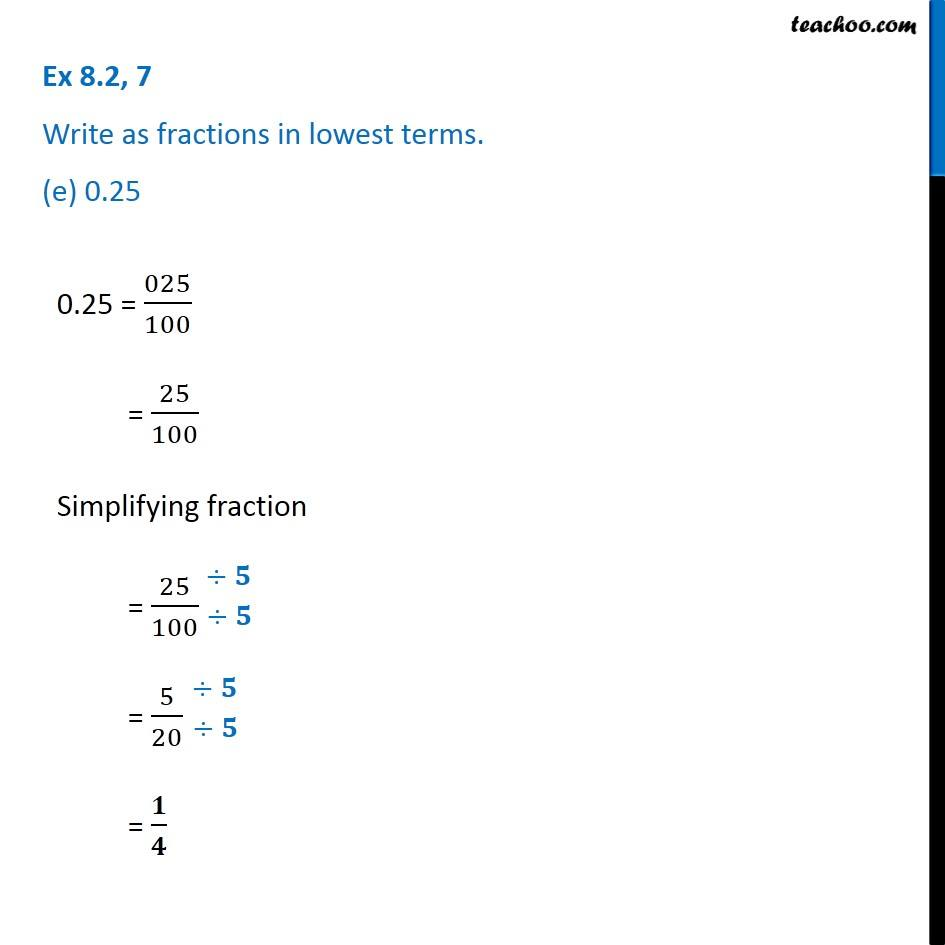1. Chapter 8 Class 6 Decimals
2. Serial order wise
3. Ex 8.2

Transcript

Ex 8.2, 7 Write as fractions in lowest terms. (e) 0.25 0.25 = 025/100 = 25/100 Simplifying fraction = 25/100 = 5/20 = 𝟏/𝟒